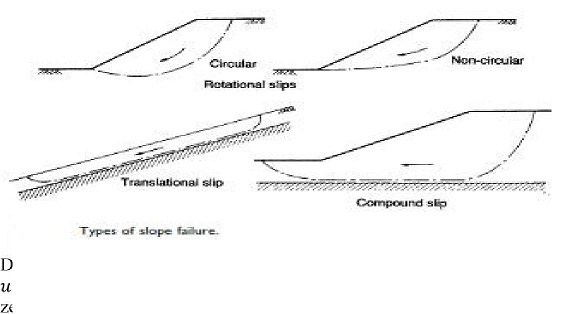Home | | Soil Mechanics | Slope Stability: Introduction

# Slope Stability: IntroductionGravitational and seepage forces tend to cause instability in natural slopes, in slopes formed by excavation and in the slopes of embankments.

SLOPE STABILITY

INTRODUCTION

Gravitational and seepage forces tend to cause instability in natural slopes, in slopes formed by excavation and in the slopes of embankments. The most important types of slope failure are illustrated in Figure. In rotational slips the shape of the failure surface in section may be a circular arc or a non-circular curve. In general, circular slips are associated with homogeneous, isotropic soil conditions and non-circular slips with non-homogeneous conditions. Translational and compound slips occur where the form of the failure surface is influenced by the presence of an adjacent stratum of significantly different strength, most of the failure surface being likely to pass through the stratum of lower shear strength. The form of the surface would also be influenced by the presence of discontinuities such as fissures and pre-existing slips. Translational slips tend to occur where the adjacent stratum is at a relatively shallow depth below the surface of the slope, the failure surface tending to be plane and roughly parallel to the slope. Compound slips usually occur where the adjacent stratum is at greater depth, the failure surface consisting of curved and plane sections. In most cases, slope stability can be considered as a two-dimensional problem, conditions of plane strain being assumed.

Design resisting moment. Characteristic values of shear strength parameters c' and tan ?should be divided by factors 1.60 and 1.25, respectively. (However, the value of c' is zero if the critical-state strength is used.) The characteristic value of parameter cu should be divided by 1.40. A factor of unity is appropriate for the self-weight of the soil and for pore water pressures. However, variable loads on the soil surface adjacent to the slope should be multiplied by a factor of 1.30.

The following limit states should be considered as appropriate:

1         Loss of overall stability due to slip failure.

2         Bearing resistance failure below embankments.

3         Internal erosion due to high hydraulic gradients and/or poor compaction.

4         Failure as a result of surface erosion.

5         Failure due to hydraulic uplift.

Excessive soil deformation resulting in structural damage to, or loss of service- ability of, adjacent structures, highways or services.

Study Material, Lecturing Notes, Assignment, Reference, Wiki description explanation, brief detail

Related Topics